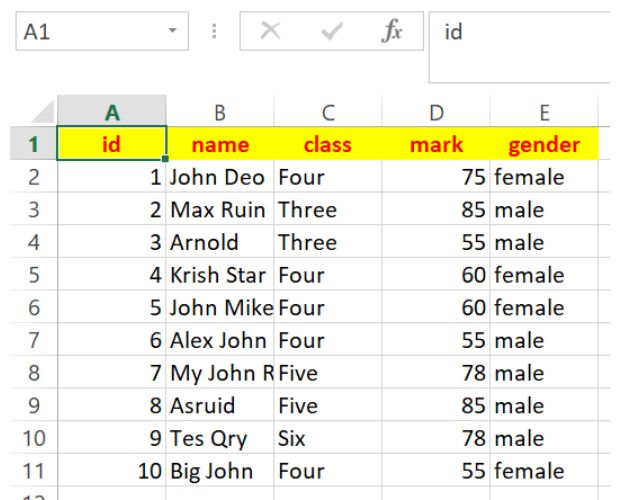# Reading Data from Excel file using openpyxl

openpyxlOpenpyxl library to read Excel file from cell or rows columns by using max min rows and columns

## Opening Excel file

``````from openpyxl import load_workbook
ws = wb['student'] # connecting to sheet

wb.close()# Close the workbook after reading``````

Here cell with row=2 and column=3 ( 1 - based index ) value is printed.
``````from openpyxl import load_workbook
ws = wb['student'] # connecting to sheet

print(ws.cell(2,3).value)# One particular cell value

wb.close()# Close the workbook after reading``````

## Row values

``iter_rows(min_row=None, max_row=None, min_col=None, max_col=None, values_only=False)``
Returns a generator
If range is not given then it starts from A1

We will get ID ( first column ) and Name( second column ) values of five records including header row
``````for data in ws.iter_rows(max_col=5,max_row=5,values_only=True):
print(data,data) # Show column 1,2 - id, name``````
Show id, name , class,mark and gender columns ( all columns ) upto 5th row.
``````for data in ws.iter_rows(max_col=5,max_row=5,values_only=True):
for d in range(len(data)):
print(data[d],end=' ') # print all data of a row
print('') # adding line break ``````
Output
``````id name class mark gender
1 John Deo Four 75 female
2 Max Ruin Three 85 male
3 Arnold Three 55 male
4 Krish Star Four 60 female``````
Remove the header and only show up to 5th row
``````for data in ws.iter_rows(min_row=2,max_col=5,max_row=6,values_only=True):
for d in range(len(data)):
print(data[d],end=' ') # print all data of a row
print('') # adding line break at end of each row ``````
Output
``````1 John Deo Four 75 female
2 Max Ruin Three 85 male
3 Arnold Three 55 male
4 Krish Star Four 60 female
5 John Mike Four 60 female``````
To get all the rows we can remove the max_rows=6 option in inter_rows()

## Column values

``iter_cols(min_col=None, max_col=None, min_row=None, max_row=None, values_only=False)``
This is not available in read only mode.

AttributeError: 'ReadOnlyWorksheet' object has no attribute 'iter_cols'

Change the mode.
``wb = load_workbook(filename='D:\student.xlsx', read_only=False)``
Getting Name column data for 6 records
``````for data in ws.iter_cols(min_row=2,min_col=2,max_row=6,max_col=2,values_only=True):
print(data)``````
Output
``('John Deo', 'Max Ruin', 'Arnold', 'Krish Star', 'John Mike')``
To include the column header ( Name ) we will remove the option `min_row=2`
``````for data in ws.iter_cols(min_col=2,max_row=6,max_col=2,values_only=True):
print(data)``````
Output
``('name', 'John Deo', 'Max Ruin', 'Arnold', 'Krish Star', 'John Mike')``

## Number of rows and columns

``````print(ws.max_column) # Max column count, output is 5
print(ws.max_row) # Max row count, output is 36``````

## Data of Last row

This can be used to generate next record id ( similar to auto incremented id column of MySQL database. )
The first column data of last row is printed here.
``print(ws.cell(ws.max_row, 1).value)``
To generate a sequence of unique numbers for List, use enumerate().

Subscribe to our YouTube Channel here

## Subscribe

* indicates required
Subscribe to plus2netplus2net.com